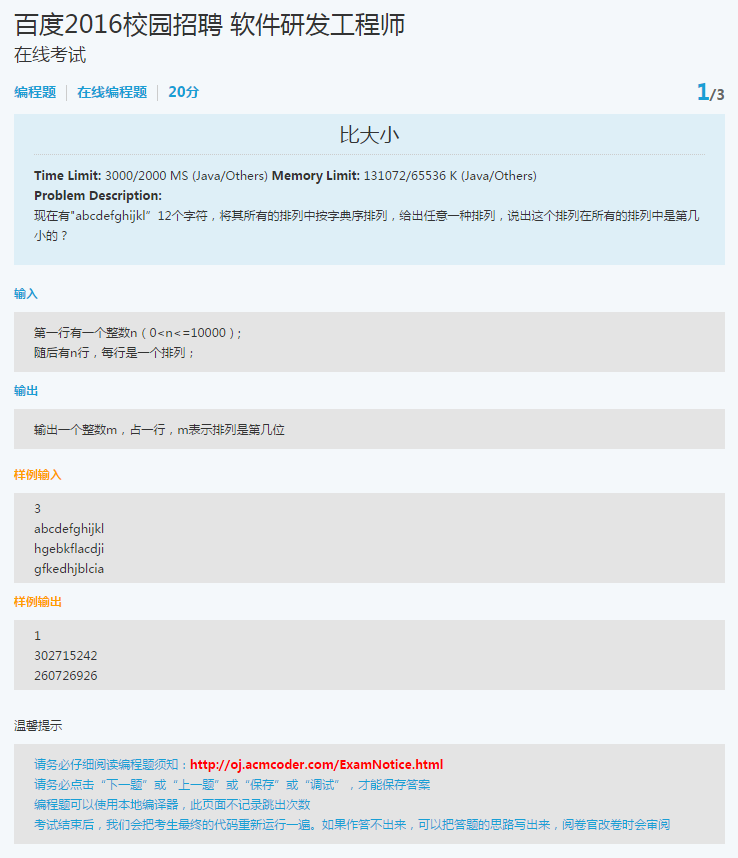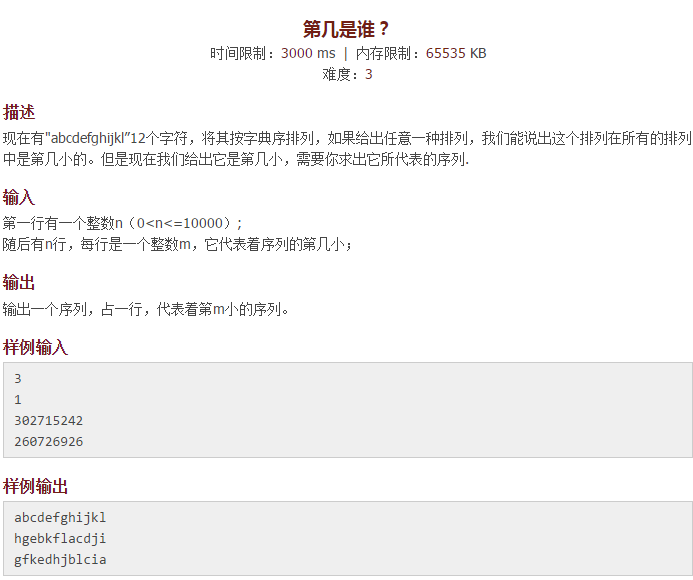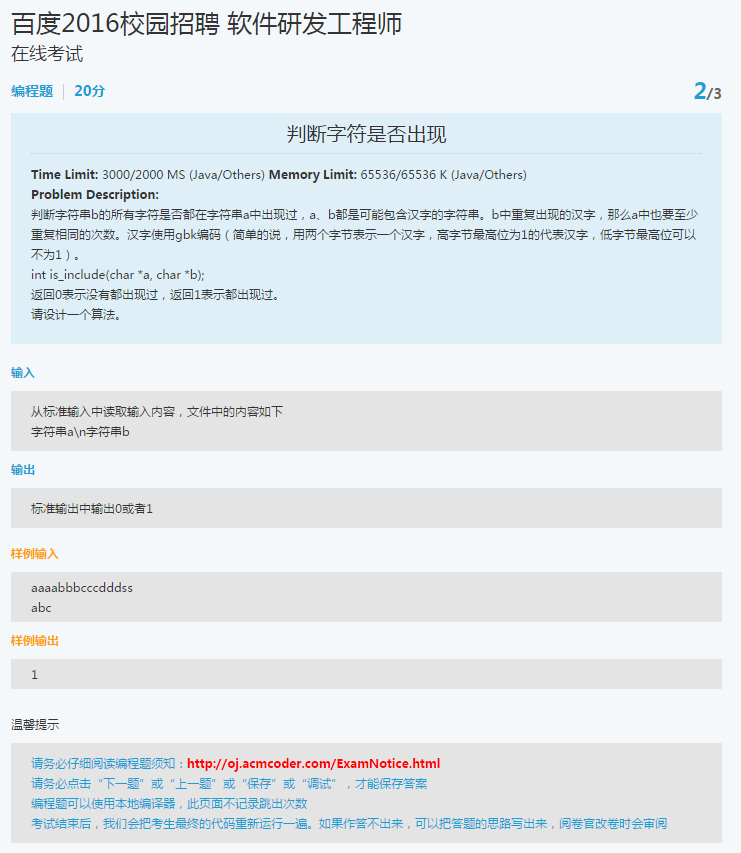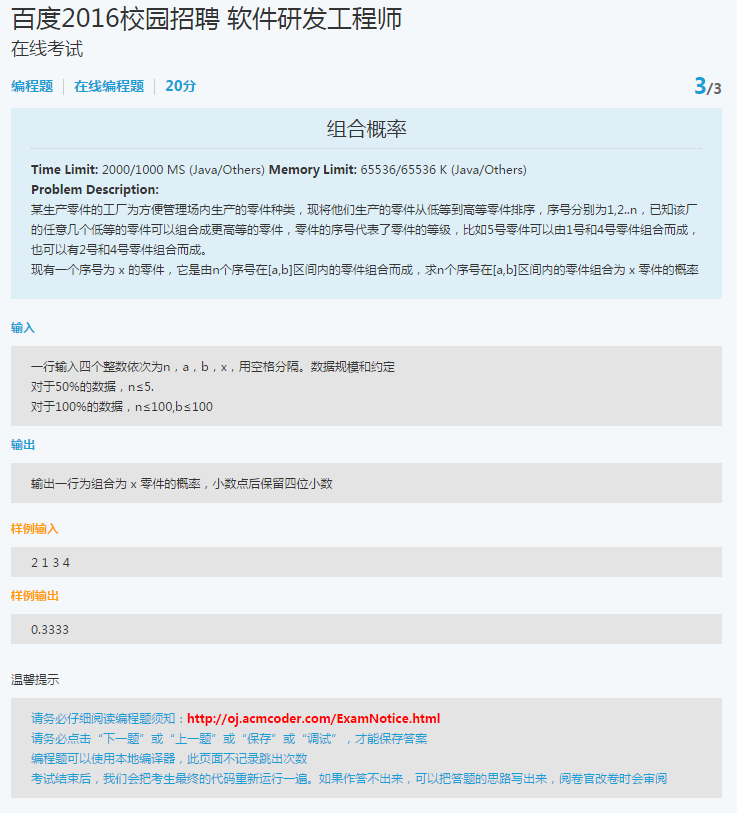### 比大小$$X=a_n*(n-1)!+a_{n-1}*(n-2)!+\cdot\cdot\cdot+a_i*(i-1)!+\cdot\cdot\cdot+a_2*(2-1)!+a_1*(1-1)!$$

$$X=a_4*3!+a_3*2!+a_2*1!+a_1*0!$$

1. 首先计算n，n等于数组S的长度，n=4
2. 再来计算a4=”b”这个元素在数组["b","c","d","a"]中是第几大的元素。”a”是第0大的元素，”b”是第1大的元素，”c”是第2大的元素，”d”是第3大的元素，所以a4=1
3. 同样a3=”c”这个元素在数组["c","d","a"]中是第几大的元素。”a”是第0大的元素，”c”是第1大的元素，”d”是第2大的元素，所以a3=1
4. a2=”d”这个元素在数组["d","a"]中是第几大的元素。”a”是第0大的元素，”d”是第1大的元素，所以a2=1
5. a1=”a”这个元素在数组["a"]中是第几大的元素。”a”是第0大的元素，所以a1=0
6. 所以X(S1)=1\*3!+1\*2!+1\*1!+0\*0!=9
7. 注意所有的计算都是按照从0开始的，如果[“a”,”b”,”c”,”d”]算为第1个的话，那么将X(S1)+1即为最后的结果

Java算法实现

### 拓展一下-求其逆过程$$X(S1)=a_4*3!+a_3*2!+a_2*1!+a_1*0!=9$$

$$1*3!+1*2!+1*1!+0*0!=9$$

$$0*3!+4*2!+1*1!+0*0!=9$$

$$0*3!+3*2!+3*1!+0*0!=9$$

$$0*3!+2*2!+5*1!+0*0!=9$$

……，但是每一个ai其实是有取值范围的，首先要知道ai表示的含义，其代表在当前剩余的序列中ai是处于第几大的位置，那么满足0<=ai<=i，同时a1必然为0，因为最后始终剩余一个元素。所以上式中只有第一个满足条件，那么a4=1，a3=1，a2=1，a1=1。推导出S1=["b","c","d","a"]

Java算法实现

### 判断字符串是否出现1. 将字符串a存储在一个map集合中，以每个字符的ASCII码作为key，以其出现的次数作为value，记为aMap
2. 遍历字符串b，对于b中的每一个字符，如果aMap的key中含有该字符的ASCII码，如果该key对应的value>1，那么将value值减1
3. 否则value=1的话，那么将该键值对从aMap中移除
4. 在判断aMap的key是否包含b中的某个字符的时候，只要有一次不包含，那么就说明没有都出现
5. 否则的话，表示b中的字符在a中都出现过

Java算法实现

### 组合概率Java算法实现